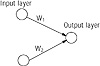# 38.C++ Neural Networks and Fuzzy Logic by Valluru B. Rao M&T Books, IDG Books Worldwide, Inc. ISBN: 1558515526   Pub Date: 06/01/95

### Instar and Outstar

Outstar and instar are terms defined by Stephen Grossberg for ways of looking at neurons in a network. A neuron in a web of other neurons receives a large number of inputs from outside the neuron’s boundaries. This is like an inwardly radiating star, hence, the term instar. Also, a neuron may be sending its output to many other destinations in the network. In this way it is acting as an outstar. Every neuron is thus simultaneously both an instar and an outstar. As an instar it receives stimuli from other parts of the network or from outside the network. Note that the neurons in the input layer of a network primarily have connections away from them to the neurons in the next layer, and thus behave mostly as outstars. Neurons in the output layer have many connections coming to it and thus behave mostly as instars. A neural network performs its work through the constant interaction of instars and outstars.

A layer of instars can constitute a competitive layer in a network. An outstar can also be described as a source node with some associated sink nodes that the source feeds to. Grossberg identifies the source input with a conditioned stimulus and the sink inputs with unconditioned stimuli. Robert Hecht-Nielsen’s Counterpropagation network is a model built with instars and outstars.

### Weights on Connections

Weight assignments on connections between neurons not only indicate the strength of the signal that is being fed for aggregation but also the type of interaction between the two neurons. The type of interaction is one of cooperation or of competition. The cooperative type is suggested by a positive weight, and the competition by a negative weight, on the connection. The positive weight connection is meant for what is called excitation, while the negative weight connection is termed an inhibition.

#### Initialization of Weights

Initializing the network weight structure is part of what is called the encoding phase of a network operation. The encoding algorithms are several, differing by model and by application. You may have gotten the impression that the weight matrices used in the examples discussed in detail thus far have been arbitrarily determined; or if there is a method of setting them up, you are not told what it is.

It is possible to start with randomly chosen values for the weights and to let the weights be adjusted appropriately as the network is run through successive iterations. This would make it easier also. For example, under supervised training, if the error between the desired and computed output is used as a criterion in adjusting weights, then one may as well set the initial weights to zero and let the training process take care of the rest. The small example that follows illustrates this point.

#### A Small Example

Suppose you have a network with two input neurons and one output neuron, with forward connections between the input neurons and the output neuron, as shown in Figure 5.2. The network is required to output a 1 for the input patterns (1, 0) and (1, 1), and the value 0 for (0, 1) and (0, 0). There are only two connection weights w1 and w2.Figure 5.2  Neural network with forward connections.

Let us set initially both weights to 0, but you need a threshold function also. Let us use the following threshold function, which is slightly different from the one used in a previous example:

 1 if x > 0 f(x) = { 0 if x ≤ 0

The reason for modifying this function is that if f(x) has value 1 when x = 0, then no matter what the weights are, the output will work out to 1 with input (0, 0). This makes it impossible to get a correct computation of any function that takes the value 0 for the arguments (0, 0).

Now we need to know by what procedure we adjust the weights. The procedure we would apply for this example is as follows.

If the output with input pattern (a, b) is as desired, then do not adjust the weights.
If the output with input pattern (a, b) is smaller than what it should be, then increment each of w1 and w2 by 1.
If the output with input pattern (a, b) is greater than what it should be, then subtract 1 from w1 if the product aw1 is smaller than 1, and adjust w2 similarly.

Table 5.9 shows what takes place when we follow these procedures, and at what values the weights settle.

step w1 w2 a b activation output comment
1 0 0 1 1 0 0 desired output is 1; increment both w’s
2 1 1 1 1 2 1 output is what it should be
3 1 1 1 0 1 1 output is what it should be
4 1 1 0 1 1 1 output is 1; it should be 0.
5 subtract 1 from w2
6 1 0 0 1 0 0 output is what it should be
7 1 0 0 0 0 0 output is what it should be
8 1 0 1 1 1 1 output is what it should be
9 1 0 1 0 1 1 output is what it should beC++ Neural Networks and Fuzzy Logic
ISBN: 1558515526
EAN: 2147483647
Year: 1995
Pages: 139

Similar book on Amazon